« Bending and shearing effect on deformations and stresses The effect of columns moment of inertia » The degree of fixity effect of columns The fixity degree of a column end, in a specific direction, depends on the relative stiffness between the column and its framing beams. The fixity degree varies from fixed to pinned. When a weak column (e.g. cross-section 300/300) is connected to strong beams (e.g. concrete beams with height 800 mm) the connection could be considered as fixed. On the contrary, when the column top is connected to a plain slab and the column base is connected to weak footing in soft soil without foundation beams, the connections could be idealised as pinned. One-end-fixed column The equivalent member of the previous column fixed at its base and pinned (or free) at its top, has the following behaviour: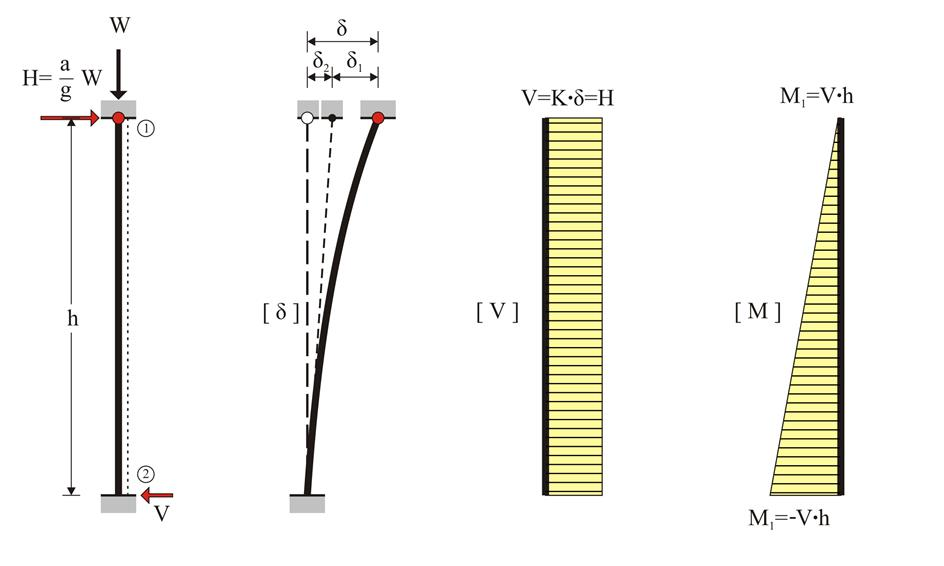Figure 5.1.2-1: One-end-fixed column: K = (3 E I / h 3) k va Figure 5.1.2-1: One-end-fixed column: K = (3 E I / h 3) k va The total displacement δ is due to: (a) the displacement δ1 due to bending caused by force H: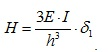(b) the displacement δ2 due to shearing caused by force H: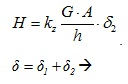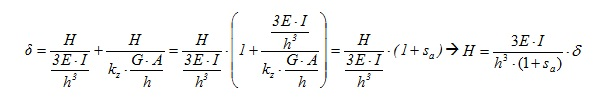The relation between the shear force H and the corresponding displacement δ is expressed as: H = K· δ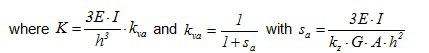The term K denotes the stiffness of the one-end-fixed column. kv ais the correction factor of the bending stiffness of the one-end-fixed column due to shearing. Always kva <1.0. It can be ignored for ordinary columns (i.e. it may be assumed that kva=1.0)while for walls kva should be taken into account. Example 5.1.2-1 Consider an one-end-fixed column with cross-section 400m m /400 mm , height h =3.0 m , elasticity modulus E =32.8 GP , bearing a concentrated mass of m =80 t at the top. The seismic acceleration design factor at top is a / g =0.10. Calculate the stress resultants and relative displacement at top. W =80 · 1000 kg · 10 m / sec 2 =800 kN , H =0.10·800=80 kN , V = H =80 kN M 1 =0 and Μ 2 =- H · h =-80·3.0=-240 kNm I =0.40·0.403/12=21.33·10-4 m 4 Without shear effect, K=3 · EI / h 3 =3 · 32.8 · 109 · Ν /m2 · 21.33 · 10-4m4/(3.03 · m3)=7.77·106 N / m . With shear effect, for G=0.5E, kz=5/6 (rectangular cross-section) and A=b · d: This expression is independent of the cross-sectional width and applies to all one-end-fixed rectangular columns.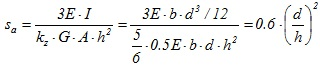For this particular column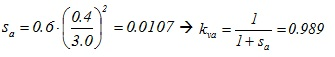therefore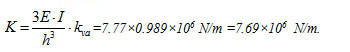In conclusion: Without shear effect, δ =H/K=80 · 103N/(7.77 · 106N/ m ) =10.30 mm . With shear effect, δ =H/K=80 · 103N/(7.69 · 106N/ m ) =10.40 mm , i.e. the displacement is 1% higher than the respective value without shear effect. Example 5.1.2-2 Consider the data and questions of the previous example for a wall with cross-section 2000/300. W =80 · 1000 kg · 10 m / sec 2 =800 kN , H =0.10·800=80 kN , V = H =80 kN M 1 =- Μ 2 =- H · h /2=-80·3.0/2=120 kNm I =0.30·2.003/12=0.20 m 4 Without shear effect, K=3 · EI / h 3 =3 · 32.8 · 109 · Ν /m2 · 0.20m4/(3.03 · m3)=728.89·106 N / m . For this particular column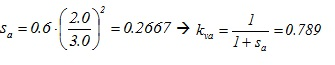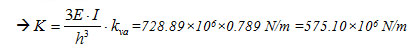Therefore without shear effect, δ =H/K=80 · 103N/(728.89 · 106N/ m )=0.110 mm . With shear effect, δ =H/K=80 · 103N/(575.10 · 106N/ m ) =0.139 mm , i.e. the displacement is 26% higher than the respective value without shear effect. Pin-ended column In the case of a column pinned at both ends, the equivalent member is not subjected to bending thus carrying neither bending moments nor shear forces. Indicatively, the case of a pin-ended column could be met at a storey without beams, at the roof and at the floor.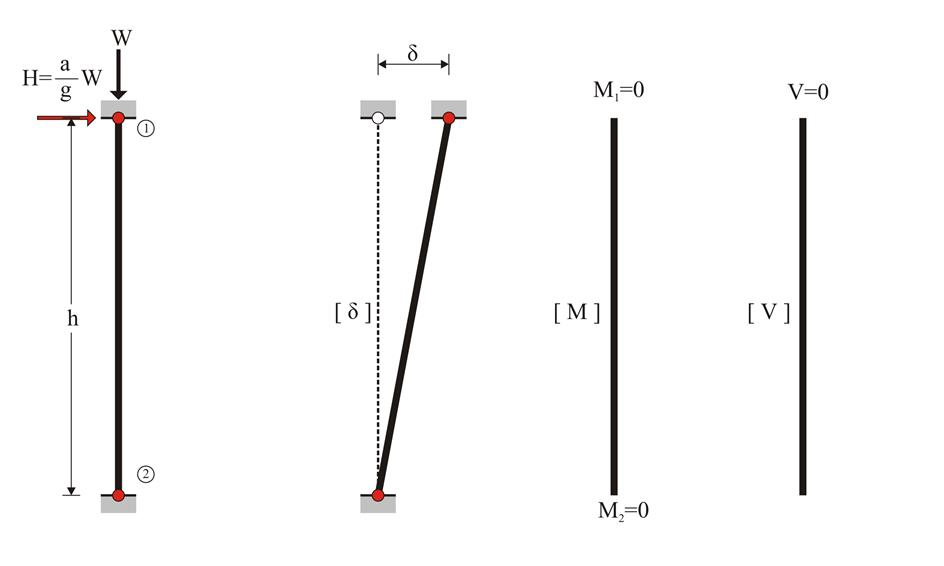Figure 5.1.2-2 Figure 5.1.2-2 Coupled columns (Frames) The three previous columns, i.e. having either both ends fixed or one end fixed or both ends pinned, are considered to compose a one-storey frame. All columns have the same material and cross-section 400/400 therefore the same E·I. The same mass at the top and therefore the same seismic load H is also applied to them. Due to the presence of the slab, the axial deformation of the frame crossbar may be practically ignored. In case of seismic action, each column top would be forced to develop identical displacement δ regardless of its stiffness.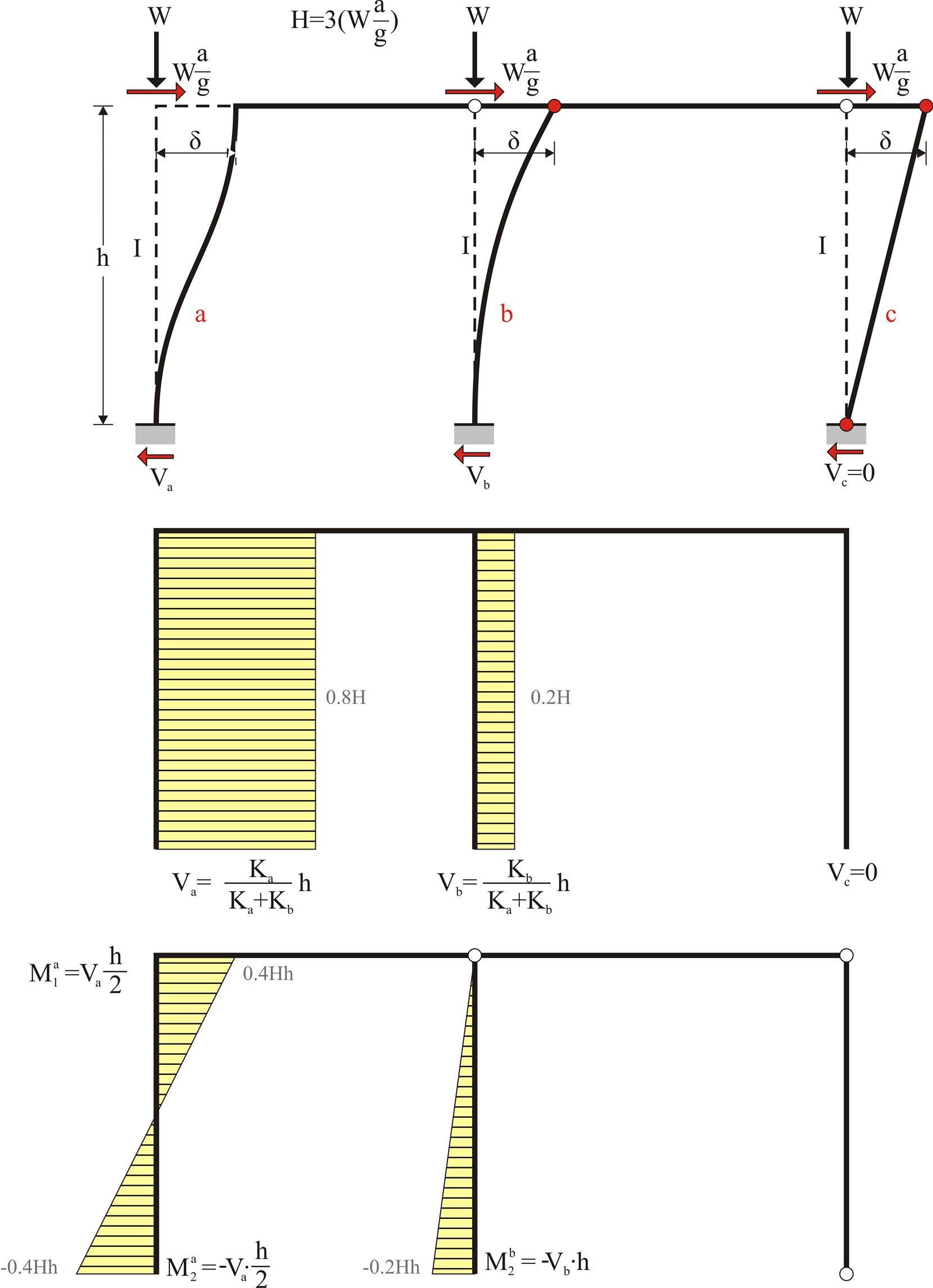Figure 5.1.2-3: 80% of the seismic force is carried by the fixed-ended column Figure 5.1.2-3: 80% of the seismic force is carried by the fixed-ended column The shear effect is considered insignificant.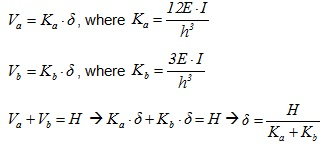Therefore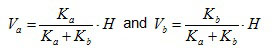Factor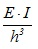is the same for all stiffnesses. So,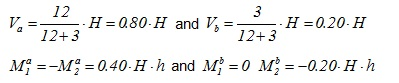It should be noted that 80% of seismic force H is carried by the first (fixed-ended) column. Example 5.1.2-3 Consider three columns identical to those of the previous examples with cross-section 400/400 and seismic acceleration factor a / g =0.10. H=3·(0.10·800kN)=240 kN Va=0.80·240=192 kN, Vb=0.20·240=48 k Ma1=-Ma2=192·3.0/2=288 kNm, Mb1=0, Mb2=-48·3.0=-144 kNm Σ( K )= Ka + Kb =15 EI / h 3 =15·32.8·109 · Ν/ m 2 · 21.33·10-4 m 4 /(3.03 · m 3 )=38.87·106 N / m δ= H /Σ( K )=240·103 N /(38.87·106 N / m ) =6.17 mm « Bending and shearing effect on deformations and stresses The effect of columns moment of inertia »